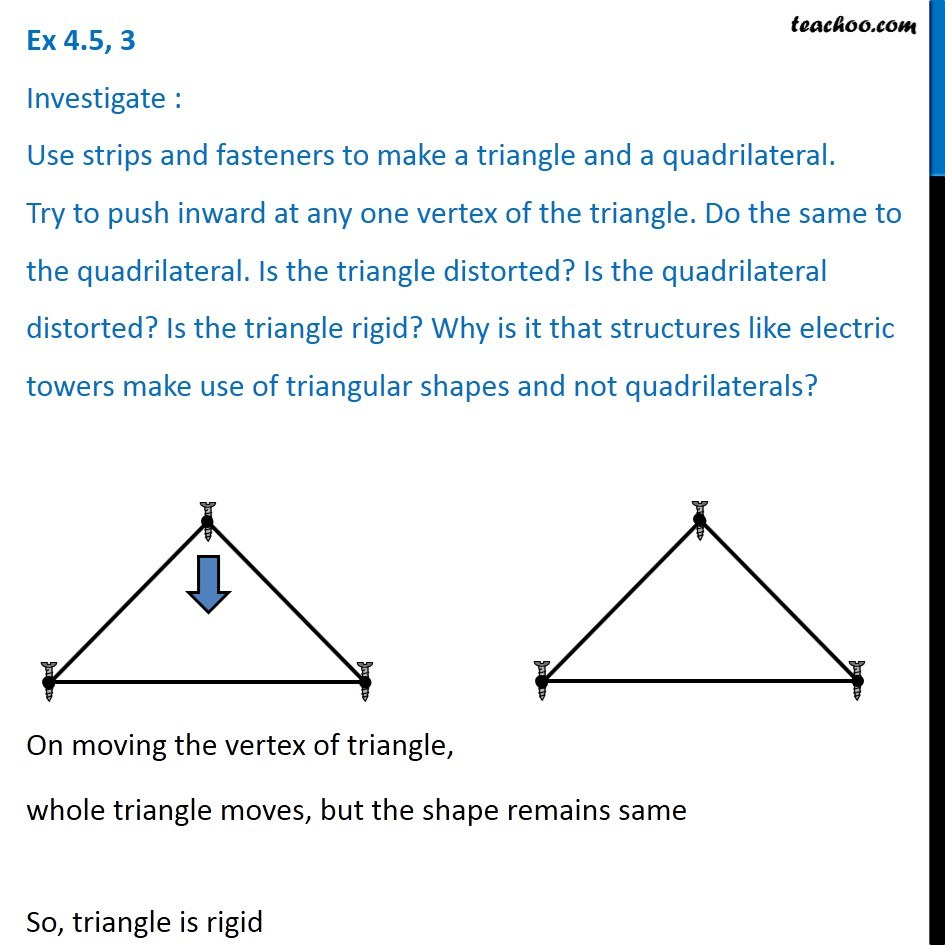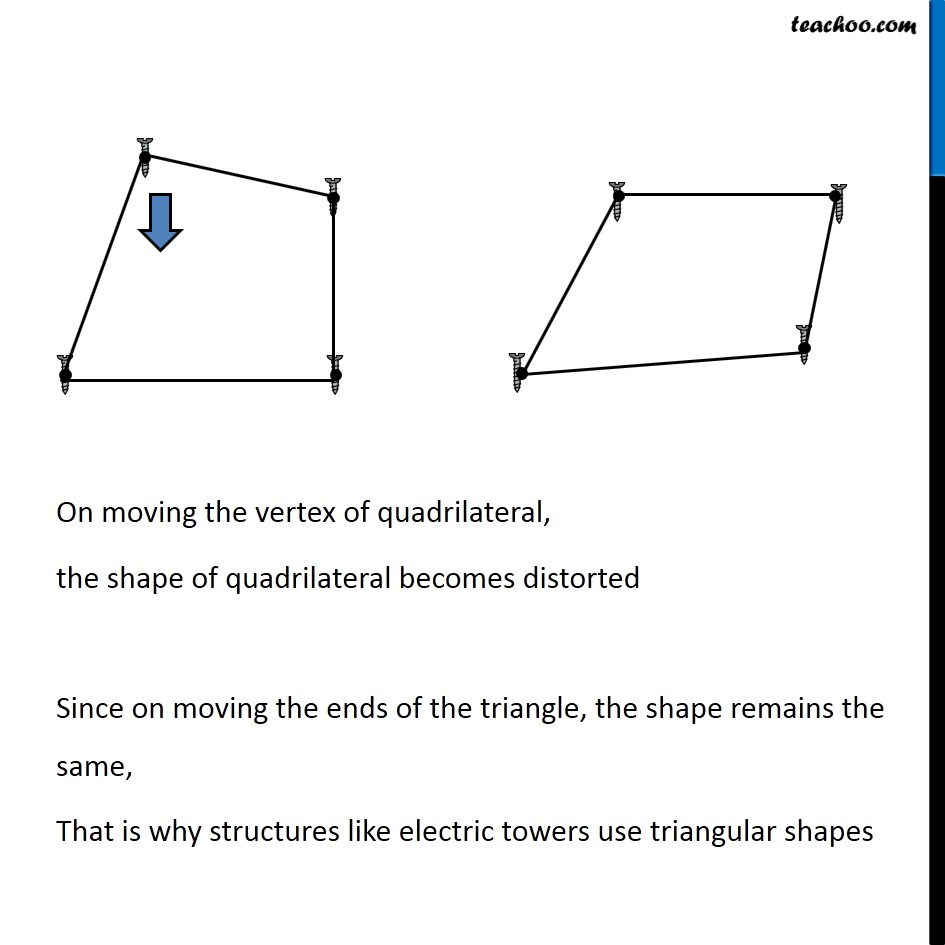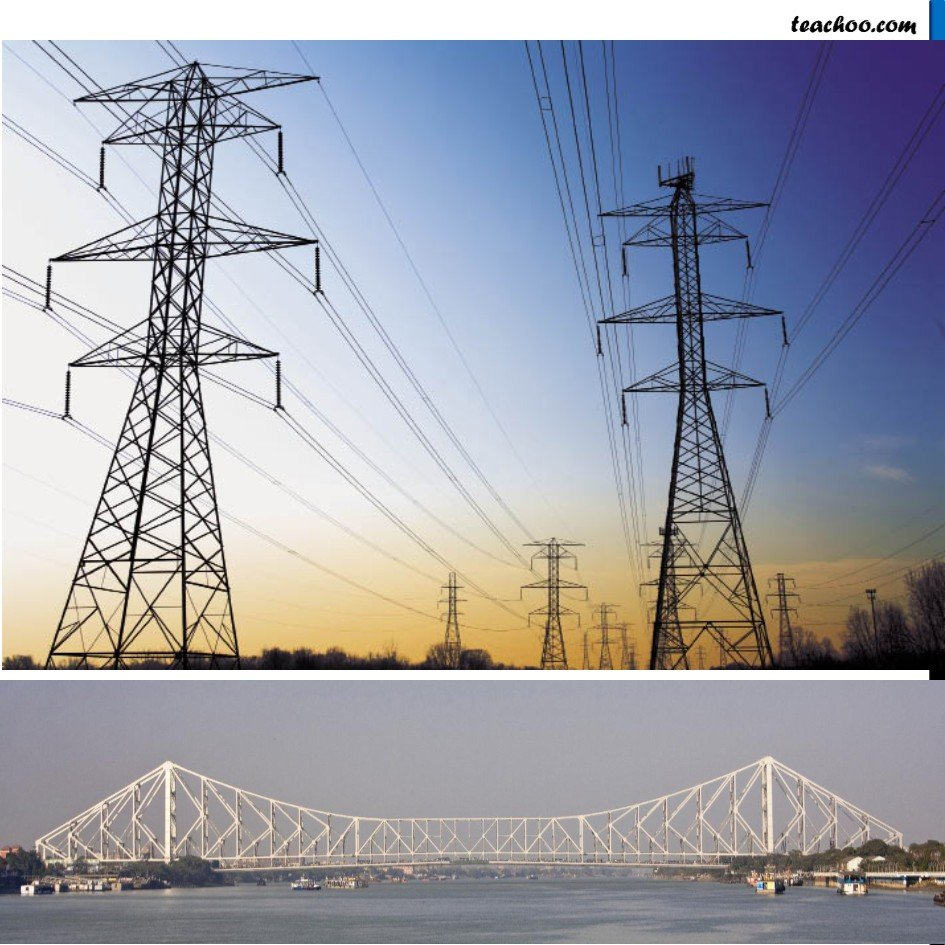Chapter 4 Class 6 Basic Geometrical Ideas
Serial order wiseLearn in your speed, with individual attention - Teachoo Maths 1-on-1 Class

### Transcript

Question 3 Investigate : Use strips and fasteners to make a triangle and a quadrilateral. Try to push inward at any one vertex of the triangle. Do the same to the quadrilateral. Is the triangle distorted? Is the quadrilateral distorted? Is the triangle rigid? Why is it that structures like electric towers make use of triangular shapes and not quadrilaterals? . . On moving the vertex of triangle, whole triangle moves, but the shape remains same So, triangle is rigid On moving the vertex of quadrilateral, the shape of quadrilateral becomes distorted Since on moving the ends of the triangle, the shape remains the same, That is why structures like electric towers use triangular shapes